### IMO Shortlist 2005 problem G3

Kvaliteta:
Avg: 3.0
Težina:
Avg: 7.0
Let$ABCD$ be a parallelogram. A variable line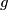$g$ through the vertex$A$ intersects the rays$BC$ and$DC$ at the points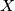$X$ and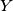$Y$, respectively. Let$K$ and$L$ be the$A$-excenters of the triangles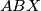$ABX$ and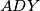$ADY$. Show that the angle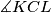$\measuredangle KCL$ is independent of the line$g$.
Source: Međunarodna matematička olimpijada, shortlist 2005